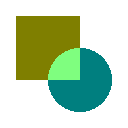# Path¶

## Path Creation¶

The MicroVG library enables the creation of vector paths composed of the following commands:

• Move
• Line
• Cubic Bezier Curve
• Close

The coordinates of the points associated with these commands can be absolute or relative.

```Path path = new Path();

path.moveTo(70, 20);
path.cubicTo(0, 0, 10, 50, 80, 90);
path.lineTo(95, 75);
path.close();
```
```Path path = new Path();

path.moveTo(70, 20);
path.cubicToRelative(-70, -20, -60, 30, 10, 70);
path.lineToRelative(15, -15);
path.close();
```

## Path Drawing¶

A path can be drawn with a call to ej.microvg.VectorGraphicsPainter.fillPath().

The drawn path will be filled with the graphic context color or with a linear gradient.

The path can be transformed by a transformation matrix (this concept is explained in Matrix section) before drawing.

A FillType and an Alpha Blending Mode can be applied during the drawing.

### Fill Path With Graphics Context Color¶

The default alpha channel value of the drawing is `0xFF` (opaque opacity).

 ```g.setColor(Colors.GRAY); VectorGraphicsPainter.fillPath(g, path, 0, 0); ```

### Fill Path With a Linear Gradient¶

The opacity value of the drawing is defined by the Alpha channel of the ARGB color values of the each linear gradient stop point.

 ```LinearGradient gradient = new LinearGradient(0, 0, 100, 0, new int[] { 0xffff0000, 0xffffff00, 0xffffffff }); VectorGraphicsPainter.fillPath(g, path, new Matrix(), gradient); ```

### Fill Type¶

A path can be drawn with a FillType argument. This argument defines the way a path will be filled.

The following values are a available:

• FillType.Winding: Specifies that “inside” is computed by a non-zero sum of signed edge crossings.
• FillType.EVEN_ODD: Specifies that “inside” is computed by an odd number of edge crossings.

 ```Path path = new Path(); path.moveTo(50, 0); path.lineTo(21, 90); path.lineTo(98, 35); path.lineTo(2, 35); path.lineTo(79, 90); path.close(); ```

### Opacity and Blending Mode¶

The opacity of the drawing can be provided to the fillPath method with a blending mode.

When the drawing is done with graphic context color, the given alpha value replaces the default value (`0xFF`).

When the drawing is done with a linear gradient, the given alpha is applied above each gradient colors alpha channel values(0x80 alpha value on `#80FFFFFF` ARGB color leads to `#40FFFFFF` color).

The supported blending modes are:

• `SRC`: The source pixels replace the destination pixels.
• `SRC_OVER`: The source pixels are drawn over the destination pixels.
• `DST_OVER`: The source pixels are drawn behind the destination pixels.
• `SRC_IN`: Keeps the source pixels that cover the destination pixels, discards the remaining source and destination pixels.
• `DST_IN`: Keeps the destination pixels that cover source pixels, discards the remaining source and destination pixels.
• `DST_OUT`: Keeps the destination pixels that are not covered by source pixels. Discards destination pixels that are covered by source pixels. Discards all source pixels.
• `PLUS`: Adds the source pixels to the destination pixels and saturates the result.
• `SCREEN`: Adds the source and destination pixels, then subtracts the source pixels multiplied by the destination.
• `MULTIPLY`: Multiplies the source and destination pixels.`SRC``SRC_OVER``DST_OVER``SRC_IN``DST_IN``DST_OUT``PLUS``SCREEN``MULTIPLY`